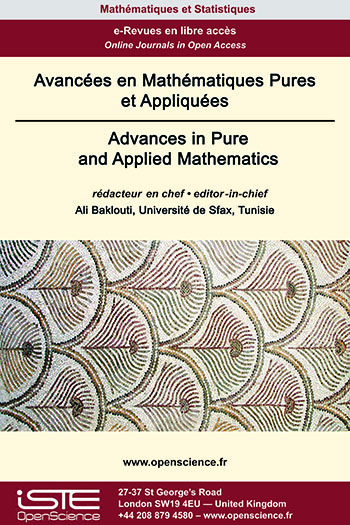# Vol 13 - Forthcoming papers

## List of Articles

[FORTHCOMING] Weyl almost automorphic functions and applications

In this paper, we reconsider the notion of a Weyl p-almost automorphic function introduced by S. Abbas  in 2012 and propose several new ways for introduction of the class of Weyl p-almost automorphic functions (1 ⩽ p < ∞). We first analyze the introduced classes of Weyl p-almost automorphic functions of type 1, jointly Weyl p-almost automorphic functions and Weyl p-almost automorphic functions of type 2 in the one-dimensional setting. After that, we introduce and analyze generalizations of these classes in the multi-dimensional setting, working with general Lebesgue spaces with variable exponents. We provide several illustrative examples and applications to the abstract Volterra integro-differential equations.

[FORTHCOMING] Global existence of solutions to the spherically symmetric Einstein-Vlasov-Maxwell system

We prove that the initial value problem with small data for the asymptotically flat spherically symmetric Einstein-Vlasov-Maxwell system admits the global in time solution in the case of the non zero shift vector. This result extends the one already known for chargeless case.

[FORTHCOMING] Existence and multiplicity of solutions for α(x)-Kirchhoff Equation with indefinite weights

In this paper, we investigate the existence of at least three weak solutions for a class of nonlocal elliptic equations with Navier boundary value conditions. The proof of our result uses the basic theory and critical point theory of variable exponential Lebesgue Sobolev spaces. Moreover a generalization of Corollary 1.1 in  is obtained.

[FORTHCOMING] Characterization of some closed linear subspaces of Morrey spaces and approximation

Let $1\leq q\leq\alpha < \infty. \left\{(L^{q}, l^{p})^{\alpha}(\mathbb{R}^d):\alpha\leq p\leq\infty \right\}$ is a nondecreasing family of Banach spaces such that the Lebesgue space is $L^{\alpha}(\mathbb{R}^d)$ its minimal element and the classical Morrey space $\mathcal{M}_{q}^{\alpha}(\mathbb{R}^d)$ is its maximal element. In this note we investigate some closed linear subspaces of $(L^{q}, l^{p})^{\alpha}(\mathbb{R}^d)$. We give a characterization of the closure in $(L^{q}, l^{p})^{\alpha}(\mathbb{R}^d)$ of the set of all its compactly supported elements and study the action of some classical operators on it. We also describe the closure in $(L^{q}, l^{p})^{\alpha}(\mathbb{R}^d)$ of the set $\mathcal{C}_{\rm{c}}^{\infty}(\mathbb{R}^d)$ of all infinitely differentiable and compactly supported functions on $\mathbb{R}^{d}$ as an intersection of other linear subspaces of $(L^{q}, l^{p})^{\alpha}(\mathbb{R}^d)$ and obtain the weak density of $\mathcal{C}_{\rm{c}}^{\infty}(\mathbb{R}^d)$ in some of these subspaces. We establish a necessary condition on a function $f$ in order that its Riesz potential $I_{\gamma}(|f|) \;(0<\gamma<1)$ be in a given Lebesgue space.### Other issues :

2020

Volume 20- 11

Issue 1 (May 2020)
Issue 2 (September 2020)

2021

Volume 21- 12

Issue 1 (January 2021)
Issue 2 (May 2021)
Issue 3 (Special AUS-ICMS 2020)
Issue 4 (September 2021)

2022

Volume 22- 13

Forthcoming papers

Issue 1 (January 2022)
Issue 2 (March 2022)
Issue 3 (June 2022)
Issue 4 (September 2022)

2023

Volume 23- 14

Issue 1 (January 2023)
Issue 2 (Special CSMT 2022)